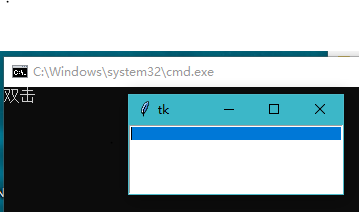python:tkinter用bind绑定双击事件不成功？

import tkinter
from tkinter import *
def p():
print('双击')
root=tkinter.Tk()
text=tkinter.Text(root,width=30,height=5)
text.pack()
text.bind("",p())
root.mainloop()2个回答life_1m 我已经是按这个贴来做的，结果不行！是不是我电脑设置问题？
4 个月之前 回复

def p():

def p(self):

text.bind("",p())

text.bind("",p)Python的tkinter模块，通过给Button添加事件绑定改变其state失败。

python tkinter中button怎么绑定多个变量

Python关于Tkinter的多线程问题

tkinter 双击 打开文件

Python tkinter图形界面BUTTON事件控制和编辑框返回值出现异常

Python中用tkinter创建GUI时，设置窗口大小，总是报错，求各位大神指导！

``` from tkinter import * root = Tk() root.title('My First Tk') root.geomtry('150x200') root.mainloop() ``` 报错信息： Traceback (most recent call last): File "G:/Lab/01jsq/jsq/main.py", line 9, in <module> root.geomtry('150x200') File "C:\Users\bitla\AppData\Local\Programs\Python\Python37-32\lib\tkinter\__init__.py", line 2101, in __getattr__ return getattr(self.tk, attr) AttributeError: '_tkinter.tkapp' object has no attribute 'geomtry' 真心不知道哪里出问题了，求各位大佬指导，跪谢！

Python_tkinter关于主窗口和弹窗的控件的显示

Python-Tkinter请问有什么办法可以在事件处理过程中，即时刷新Text的信息？

python3 tkinter Entry组件获取输入内容，通过button组件运行失败

![图片说明](https://img-ask.csdn.net/upload/202003/21/1584762096_611414.png) 如图我已经触发了Start按钮，且按照项目要求Start按钮触发的程序要持续运行，那么此时我应该如何触发Quit按钮？ ``` def start(): cap = cv2.VideoCapture(0) detector = dlib.get_frontal_face_detector() predictor = dlib.shape_predictor('F:\\shape_predictor_68_face_landmarks.dat\\shape_predictor_68_face_landmarks.dat') while True: ret, frame = cap.read() dets = detector(frame, 1) if len(dets) > 0: for i, d in enumerate(dets): x, y, w, h = d.left(),d.top(),d.right(),d.bottom() cv2.rectangle(frame, (x - 10, y - 10), ( w + 10, h + 10), (0, 255, 0), 2) shape = predictor(frame, d) for index, pt in enumerate(shape.parts()): pt_pos = (pt.x, pt.y) cv2.circle(frame, pt_pos, 1, (255, 0, 0), 2) font = cv2.FONT_HERSHEY_SIMPLEX cv2.putText(frame, str(index+1),pt_pos,font, 0.3, (0, 0, 255), 1, cv2.LINE_AA) cv2.imshow('frame', frame) cap.release() cv2.destroyAllWindows() ```

python使用tkinter调用新窗口，被调用的窗口点击右上角关闭按钮会报错，怎么屏蔽按钮

python tkinter如何与线程通讯？

python3 tkinter获取Entry输入值报错

``` #!/usr/bin/env python # encoding: utf-8 from tkinter import * top = Tk() top.title('计算器') top.grid_size() label0 = Label(top, text='计算器', font='仿宋 24 bold', width=20, height=2) label0.grid(row=0, column=1, columnspan=3, rowspan=2) label1 = Label(top, text='输入参数一：', font='楷体 13', width=16, height=1) label1.grid(row=2, column=0) label2 = Label(top, text='输入参数二：', font='楷体 13', width=16, height=1) label2.grid(row=3, column=0) input1 = Entry(top, width=20) data1 = int(input1.get()) input1.grid(row=2, column=1) input2 = Entry(top, width=20) data2 = int(input2.get()) input2.grid(row=3, column=1) button1 = Button(top, text='滚加', width=20, height=1) button1.grid(row=6, column=0) jieguo = Text(top, width=40, height=6) jieguo.grid(row=2, column=2, rowspan=4, columnspan=2) button6 = Button(top, text='退出', command=top.quit(), width=110) button6.grid(row=8, column=0, columnspan=5) mainloop() ``` 做的一个小计算器，具体计算过程还没写，刚写了一个可视界面，运行时报错，问问各位大神，(=@__@=)哪里错了 data1 = int(input1.get()) ValueError: invalid literal for int() with base 10: ''

python中tkinter自带的下载文件进度条

python2 中tkinter 打开文件 中文乱码

python中用tkinter加email写邮件发送系统

python用tkinter写的记事本,连续查找字符串函数,运行程序,结果能出来,但是窗口也死了

def find_char(self,event = None): target = simpledialog.askstring("简易文本编辑器","寻找字符串") if target: end = self.st.index(tk.END) print(end) endindex = end.split(".") end_line = int(endindex) end_column = int(endindex) pos_line =1 pos_column=0 length =len(target) while pos_line <= end_line : if pos_line == end_line and pos_column +length > end_column: break elif pos_line < end_line and pos_column + length >500: pos_line = pos_line + 1 pos_column = (pos_column + length) -500 if pos_column > end_column: break else: pos = str(pos_line)+"."+str(pos_column) where = self.st.search(target,pos,tk.END) if where: print(where) where1 =where.split(".") sele_end_col = str(int(where1)+length) sele = where1 + "."+ sele_end_col self.st.tag_add(tk.SEL,where,sele) self.st.mark_set(tk.INSERT,sele) self.st.see(tk.INSERT) self.st.focus() again = messagebox.askokcancel(title = "继续查询么") if again: pos_line = int(where1) pos_column = int(sele_end_col) else: aa=messagebox.showinfo(title = "你终于还是放弃了我",message = "你放弃了我--!") if aa: sys.exit()

Java基础知识面试题（2020最新版）

Intellij IDEA 实用插件安利

1. 前言从2020 年 JVM 生态报告解读 可以看出Intellij IDEA 目前已经稳坐 Java IDE 头把交椅。而且统计得出付费用户已经超过了八成（国外统计）。IDEA 的...

MySQL数据库面试题（2020最新版）

2020阿里全球数学大赛：3万名高手、4道题、2天2夜未交卷

HashMap底层实现原理，红黑树，B+树，B树的结构原理 Spring的AOP和IOC是什么？它们常见的使用场景有哪些？Spring事务，事务的属性，传播行为，数据库隔离级别 Spring和SpringMVC，MyBatis以及SpringBoot的注解分别有哪些？SpringMVC的工作原理，SpringBoot框架的优点，MyBatis框架的优点 SpringCould组件有哪些，他们...

《Oracle Java SE编程自学与面试指南》最佳学习路线图2020年最新版（进大厂必备）